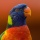Home
IT Knowledge
Inspiration
Languages
EN

# Python - assign string to variable

0 points
Created by:DoLLot
244

In this article, we would like to show you how to assign string to a variable in Python.

Quick solution:

``````my_string = 'ABC'
print(my_string)``````

## Practical example

In this example, we use single quotation marks (`''`) to assign string to a variable in Python. You can also use double quotation marks (`""`).

``````my_string = 'ABC'
print(my_string)``````

Output:

``ABC``

## Multiline string

In this example, we use three single quotation marks to assign string to a variable in Python. You can also use three double quotation marks.

``````my_string = '''line1
line2
line3'''

print(my_string)``````

Output:

``````line1
line2
line3``````